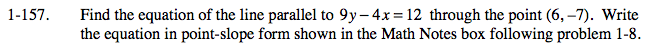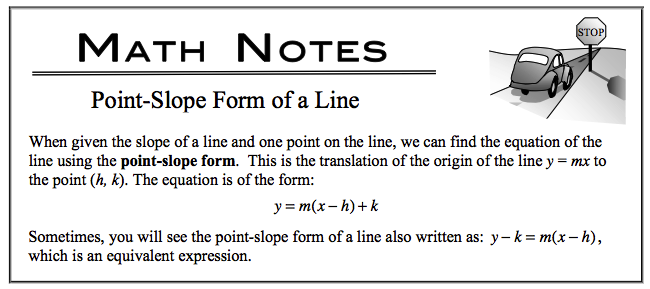### Home > CALC > Chapter 1 > Lesson 1.4.2 > Problem1-157

1-157.

Find the equation of the line parallel to 9y − 4x = 12 through the point (6, −7). Write the equation in point-slope form shown in the Math Notes box following problem 1-8. Homework Help ✎Since parallel lines have the same slope, find the slope by rearranging the equation so that y = ____________.

To convert to point-slope, see the notes below: